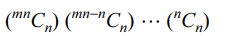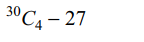## Permutations and Combinations Questions and Answers Part-9

1. Consider all 2n – 1 non-empty subsets of the set{1, 2, 3, .... n } product of each of its elements. Sum of all these products is
a) (n + 1)!
b) (n + 1)! + 1
c) (n + 1)! – 1
d) n! – 2

Explanation: Consider the product (1 + 1) (1 + 2) .... (1 + n)

2. Consider all $2^{n}-1$  non-empty subsets of the set {1, 2, .... , n} . For every such subset we find the product of reciprocals of each of its elements. Sum of all these products is
a) n
b) n + 1
c) n!
d) n!-1

Explanation:3. The number of ways in which we can arrange 4 letters of the word MATHEMATICS is given by
a) 136
b) 2454
c) 1680
d) 192

Explanation:4. The number of ways in which we can distribute mn students equally among m sections is given by
a) $\frac{\left(mn\right)!}{n!}$
b) $\frac{\left(mn\right)!}{\left(n!\right)^{m}}$
c) $\frac{\left(mn\right)!}{m!n!}$
d) $\left(mn\right)^{m}$

Explanation:5. If a polygon has 90 diagonals, the number of its sides is given by
a) 12
b) 11
c) 10
d) 15

Explanation:6. Out of 10 white, 8 black and 6 red balls, the number of ways in which one or more balls can be selected is given by
a) 681
b) 691
c) 679
d) 692

Explanation: (10 + 1) (8 + 1) (6 + 1) - 1

7. A is a set containing n elements. A subset P of A is chosen. The set A is reconstructed by replacing the elements of P. A subset Q of A is again chosen. The number of ways of choosing P and Q so that $P\cap Q$   contains exactly two elements is
a) $9\times ^{n}C_{2}$
b) $3^{n}- ^{n}C_{2}$
c) $2\times ^{n}C_{n}$
d) $^{n}C_{2}.3^{n-2}$

Explanation:8. Ten different letters of an alphabet are given. Words with five letters are formed from these given letters . The number of words which have at least one of their letters repeated is
a) 69760
b) 30240
c) 99748
d) 60480

Explanation:9. The number of ways in which we can select four numbers from 1 to 30 so as to exclude every selection of four consecutive numbers is
a) 27378
b) 27405
c) 27397
d) 1905010. If $^{n}C_{4}$ , $^{n}C_{5}$ and $^{n}C_{6}$ are in A.P., the value of n can be